Related Articles
Reciprocal of Trigonometric Ratios
• Last Updated : 23 Mar, 2021

Trigonometry is all about triangles or to be more precise about the relation between the angles and sides of a right-angled triangle. In this article, we will be discussing about the ratio of sides of a right-angled triangle with respect to its acute angle called trigonometric ratios of the angle and find the reciprocals of these Trigonometric Ratios.

Consider the following triangle: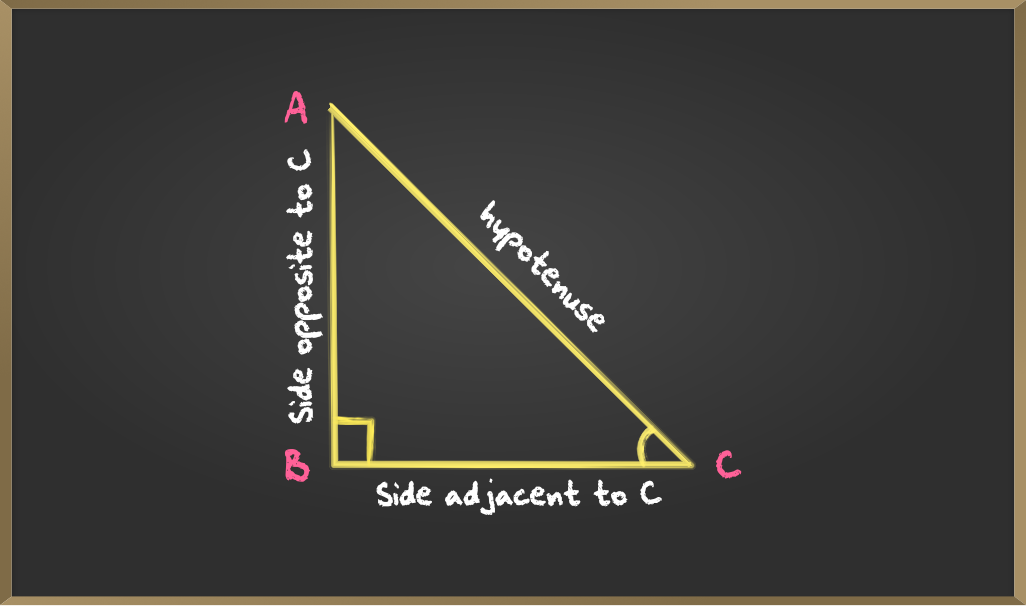Some basic points to remember

• The ∠XYZ is just called ∠Y,  example ∠ACB is just called ∠C
• The side which is opposite to an ∠θ (θ is any acute angle)  is called the opposite side with respect to ∠θ
• The side which is adjacent to an ∠θ is called the adjacent side with respect to ∠θ
• The longest side of a right-angled triangle is the hypotenuse.

## Basic Trigonometric ratios

The trigonometric ratios of an acute angle in a right triangle are the relationship between the angle and the length of two sides. Here we will use the angle C in  △ABC to define all the trigonometric ratios. The ratios defined below are abbreviated as sin C, cos C, and tan C respectively.

A. Sine: Sine of ∠C is the ratio between BA and AC that is the ratio between the side opposite to ∠C and the hypotenuse.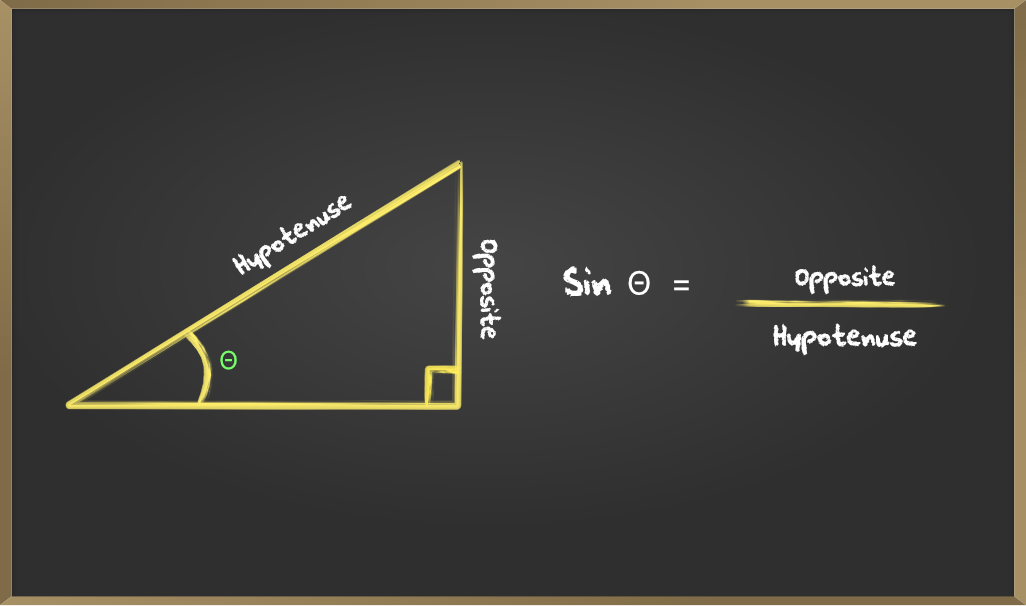B. Cosine: Cosine of ∠C is the ratio between BC and AC that is the ratio between the side adjacent to ∠C  and the hypotenuse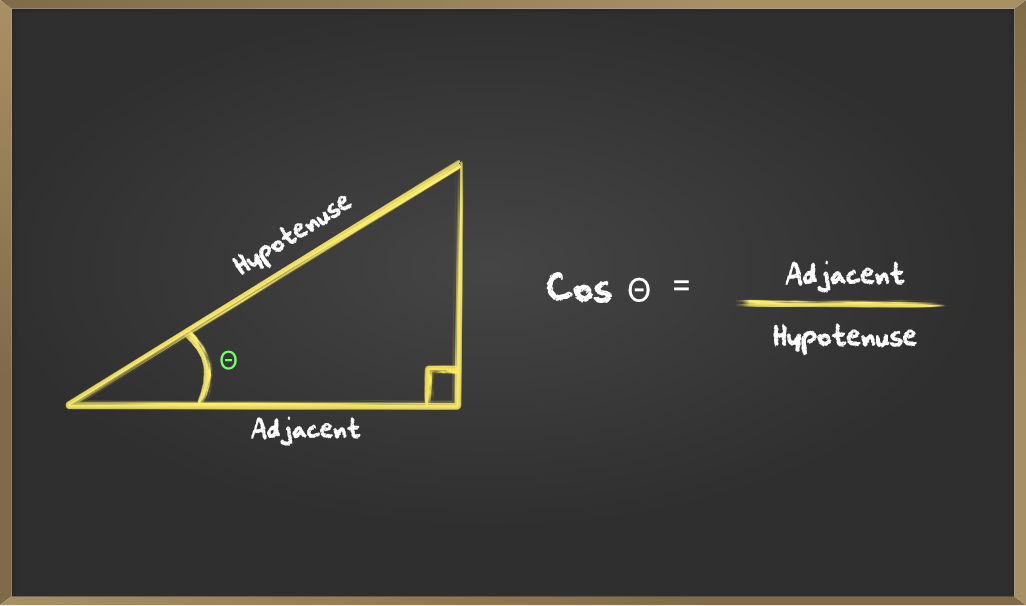C. Tangent: Tangent of ∠C is the ratio between BA and BC that is the ratio between the side opposite and adjacent to ∠C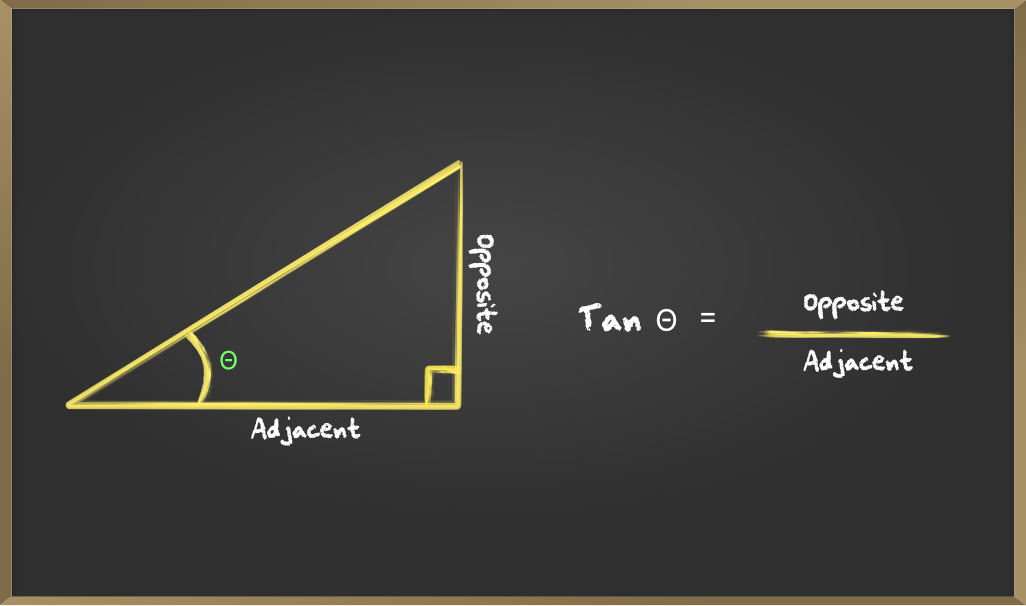## Reciprocals of Trigonometric Ratios

Reciprocals of basic trigonometric ratios are the inverse values of the sin, cos, and tan values that are computed by reciprocating the sides required for computing the ratio. You will see that cosec A, sec A, and cot A are respectively, the reciprocals of sin A, cos A, and tan A from the following diagrams and examples.

### A. Reciprocal of sin C

Sine is the ratio of the opposite side to the Hypotenuse. cosecant is the reciprocal of sin that is the ratio between the hypotenuse and the opposite side.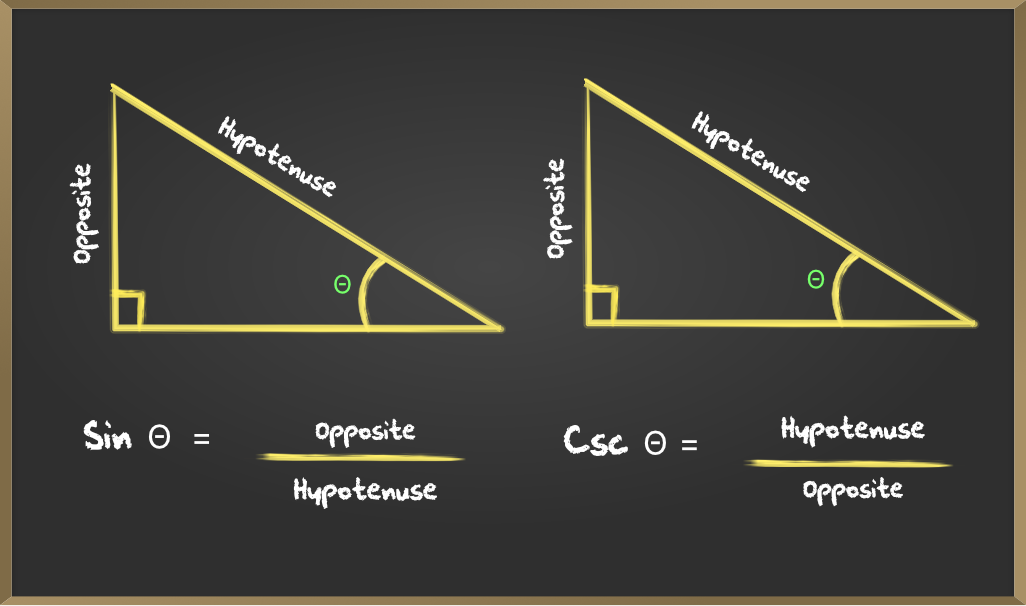#### Example 1: If the value of sin x = 0.47 then find the value of cosec x?

Solution: Value of sin x = 0.47

andExample 2: If the value of cosec C = 3 then find the value of sin C?

Solution: Value of cosec C = 4### B. Reciprocal of cos C

Cos is the ratio of the adjacent side to the Hypotenuse. secant is the reciprocal of cos that is the ratio between the hypotenuse and the adjacent side.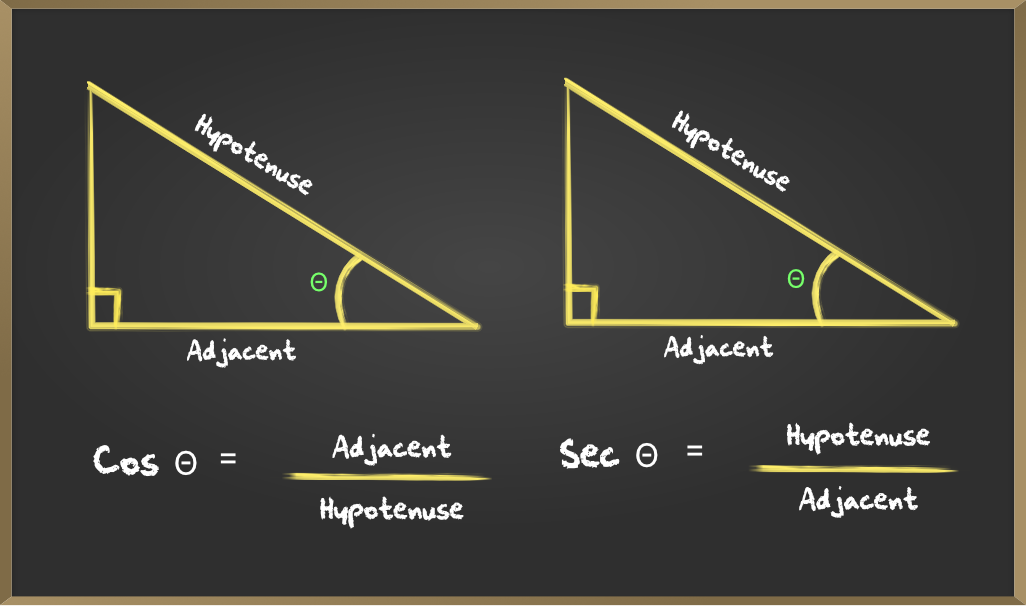#### Example 1: If the value of cos x = 0 then find the value of sec x?

Solution: cos x = 0sec x is not defined as division by 0 is not possible

Example 2: If the value of sec x = 100 then find the value of cos x?

Solution: sec x = 100### C. Reciprocal of tan C

Tan is the ratio of the opposite side to the Adjacent side. cotangent is the reciprocal of tan that is the ratio between the adjacent side and the opposite side.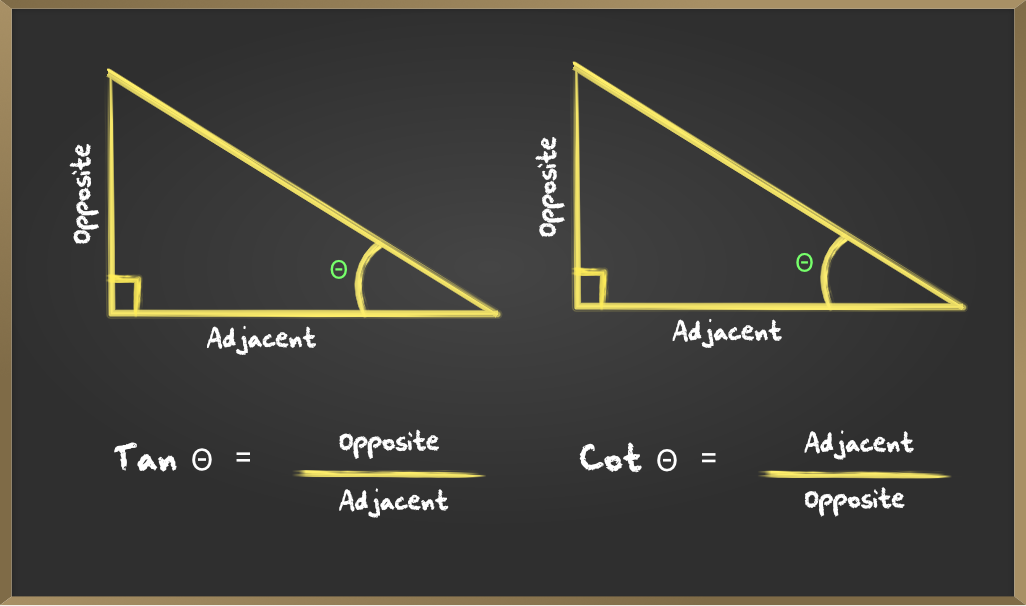#### Example 1: Find the value of tan x and cot x if x = 30°?

Solution: x = 30°Example 2: If the value of tan x = 5 find the value of cot x?

Solution: tan x = 5My Personal Notes arrow_drop_up